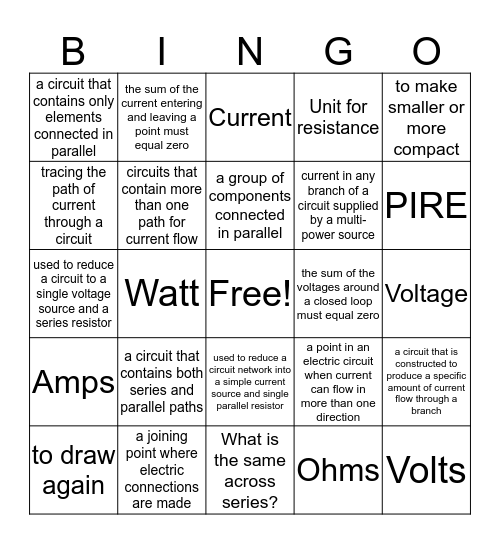# Unit 8 & 9 Key TermsThis bingo card has a free space and 24 words: a circuit that contains both series and parallel paths, a joining point where electric connections are made, a group of components connected in parallel, to draw again, to make smaller or more compact, a circuit that contains only elements connected in parallel, tracing the path of current through a circuit, the sum of the current entering and leaving a point must equal zero, the sum of the voltages around a closed loop must equal zero, used to reduce a circuit network into a simple current source and single parallel resistor, current in any branch of a circuit supplied by a multi-power source, used to reduce a circuit to a single voltage source and a series resistor, a point in an electric circuit when current can flow in more than one direction, a circuit that is constructed to produce a specific amount of current flow through a branch, circuits that contain more than one path for current flow, Ohms, Voltage, Current, PIRE, Watt, Volts, Amps, Unit for resistance and What is the same across series?.

## Play Online